# Types of Elasticity of Demand

The elasticity of demand measures the relative change in the total amount of goods or services that are demanded by the market or by an individual. The quantity demanded depends on several factors. Some of the most important factors are the price of the good or service, the price of other goods and services, the income of the population or person and the preferences of the consumers.

So, we have several types of elasticity of demand according to the source of the change in the demand. For example, if the price is the source of the change, we have the “price elasticity of demand”.

According to the source of the change, the following types of elasticity of demand can be mentioned:

1. Price Elasticity of Demand
2. Cross Elasticity of Demand (the elasticity in relation to the change of the price of other good and services)
3. Income Elasticity of Demand

According to the degree of the change in the demand, the elasticity can be classified in:

1. Perfectly Elastic
2. Relatively Elastic
3. Unit Elasticity
4. Relatively Inelastic
5. Perfect Inelastic

## Price Elasticity of DemandThe price elasticity of demand is the proportional change in the quantity demanded, relative to the proportional change in the price of the good.

Price elasticity of demand = Percentage change in quantity demanded / percentage change in price = ΔQ/Q / ΔP/P

## Cross Elasticity of Demand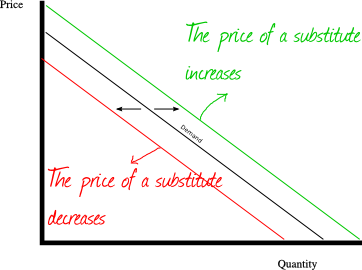The cross elasticity of demand is the proportional change in the quantity demanded, relative to the proportional change in the price of another good.

Price elasticity of demand = Percentaje change in quantity demanded / percentaje change in price of another good = ΔQ1/Q1 / ΔP2/P2

Looking at the chart, the change in the price of another good shifts the demand curve to the left or to the right.

If the two goods are substitutes, the cross elasticity of demand is positive.
If the two goods are complements, the cross elasticity of demand is negative.

## Income Elasticity of Demand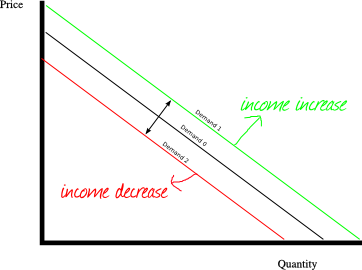The income elasticity of demand is the proportional change in the quantity demanded, relative to the proportional change in the income.

Income elasticity of demand = Percentaje change in quantity demanded / percentaje change in the income = ΔQ/Q / ΔI/I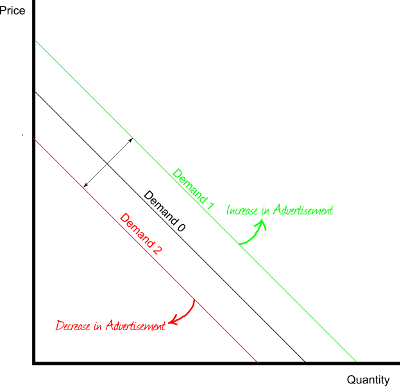The advertisement elasticity of demand is the proportional change in the quantity demanded, relative to the proportional change in the price of another good.

## According to the value of the elasticity

Up to here, we have pointed out different types of elasticity according to the function we are analyzing, and according to the inputs we are considering. Now we will see how the supply and the demand can be classified according to the value of the elasticity.

## Perfectly Elastic Demand: EP → -∞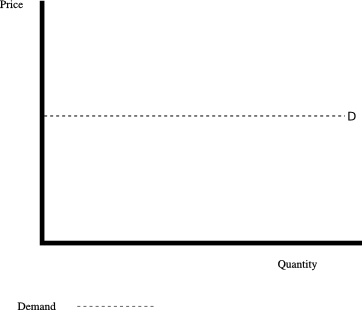Perfect Elastic Demand: The elasticity tends towards -∞.

When the demand is perfect elastic, it drops to zero in the face of a minimal price increase. If the price is the same of below the point where the demand touches the vertical axis, the market will demand all the quantity offered.

## Relatively Elastic Demand: -∞ < EP < -1, unit elasticity demand and relatively inelastic demand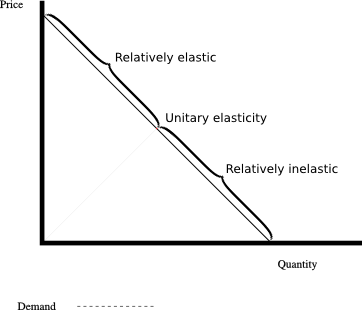Relatively elastic demand: The elasticity is between -1 and -∞.
Unit Elasticity Demand: EP = -1 , the elasticity is -1.
Relatively Inelastic Demand: -1 < EP < 0 , the elasticity is between 0 and -1.

## Perfect inelastic demand: EP = 0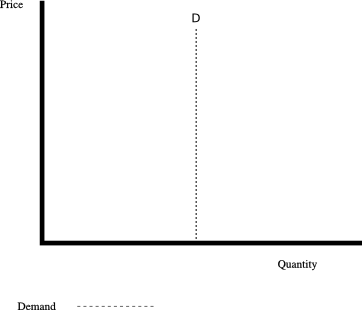A perfect inelastic demand has an elasticity of 0. The quantity demanded will not change despite changes in the price.